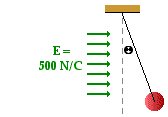# Electricity: Static Electricity

## Static Electricity: Audio Guided Solution

#### Problem 28:A thread is tied to a 2.50-gram pith ball and attached to the ceiling at a location of a uniform electric field with an intensity of 5.00x102 N/C. The pith ball experiences a force which causes it to deflect from an otherwise vertical alignment by an angle of 12.6° from the vertical. Use a free-body diagram and electrostatic principles to perform the following calculations.
a. Determine the force of gravity acting upon the pith ball.
b. Determine the vertical component of the tension force of the thread.
c. Determine the horizontal component of the tension force of the thread.
d. Determine the quantity of charge on the pith ball.
e. What type of charge - positive or negative - does the pith ball possess?

## Audio Guided Solution

Click to show or hide the answer!

## Habits of an Effective Problem Solver

• Read the problem carefully and develop a mental picture of the physical situation. If necessary, sketch a simple diagram of the physical situation to help you visualize it.
• Identify the known and unknown quantities; record them in an organized manner. A diagram is a great place to record such information. Equate given values to the symbols used to represent the corresponding quantity - e.g., Q1 = 2.4 μC; Q2 = 3.8 μC; d = 1.8 m; Felect = ???.
• Use physics formulas and conceptual reasoning to plot a strategy for solving for the unknown quantity.
• Identify the appropriate formula(s) to use.
• Perform substitutions and algebraic manipulations in order to solve for the unknown quantity.